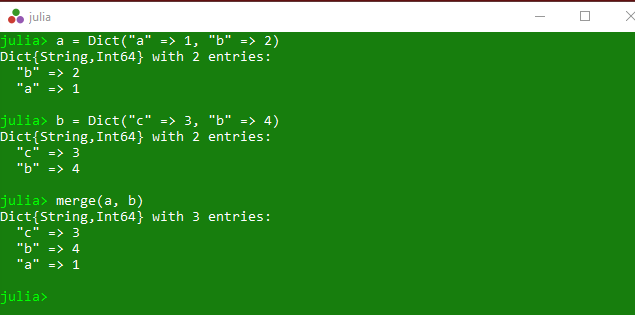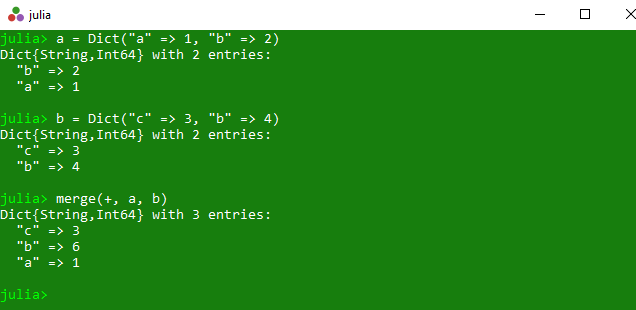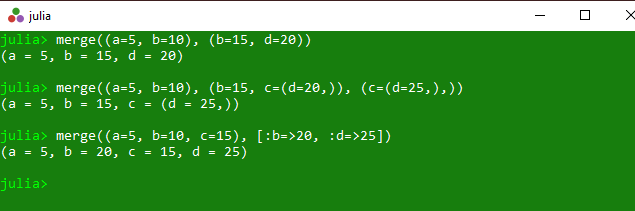Related Articles

# Merging Dictionary collections in Julia – merge() and merge!() Methods

• Last Updated : 26 Mar, 2020

The `merge()` is an inbuilt function in julia which is used to construct a merged collection from the specified collections.

Syntax:
merge(d::AbstractDict, others::AbstractDict…)
or
merge(combine, d::AbstractDict, others::AbstractDict…)
or
merge(a::NamedTuple, bs::NamedTuple…)

Parameters:

• d::AbstractDict: First specified collection.
• others::AbstractDict: Second specified collection.
• combine: Specified combing operation.
• NamedTuple: Specified different tuples..

Returns: It returns the merged collection.

Example 1:

 `# Julia program to illustrate ``# the use of merge() method`` ` `# Getting the the merged collection.``a ``=` `Dict``(``"a"` `=``> ``1``, ``"b"` `=``> ``2``)``b ``=` `Dict``(``"c"` `=``> ``3``, ``"b"` `=``> ``4``)``prinln(merge(a, b))`

Output:Example 2:

 `# Julia program to illustrate ``# the use of merge() method`` ` `# Getting the the merged collection``# along with specified operation ``# over the key's value``a ``=` `Dict``(``"a"` `=``> ``1``, ``"b"` `=``> ``2``)``b ``=` `Dict``(``"c"` `=``> ``3``, ``"b"` `=``> ``4``)``prinln(merge(``+``, a, b))`

Output:Example 3:

 `# Julia program to illustrate ``# the use of merge() method`` ` `# Getting the the merged tuple``println(merge((a ``=` `5``, b ``=` `10``), (b ``=` `15``, d ``=` `20``)))``println(merge((a ``=` `5``, b ``=` `10``), (b ``=` `15``, c ``=``(d ``=` `20``, )), (c ``=``(d ``=` `25``, ), )))`` ` `# Merging tuple of key-value pairs with``# iteration operation``println(merge((a ``=` `5``, b ``=` `10``, c ``=` `15``), [:b ``=``>``20``, :d ``=``>``25``]))`

Output:#### merge!()

The `merge!()` is an inbuilt function in julia which is used to update collection with pairs from the other collections.

Syntax:
merge!(d::AbstractDict, others::AbstractDict…)
or
merge!(combine, d::AbstractDict, others::AbstractDict…)

Parameters:

• d::AbstractDict: First specified collection.
• others::AbstractDict: Second specified collection.
• combine: Specified combing operation.

Returns: It returns the updated collection with pairs from the other collections.

Example 1:

 `# Julia program to illustrate ``# the use of merge !() method`` ` `# Getting the updated collection with``# pairs from the other collections.``A ``=` `Dict``(``"a"` `=``> ``1``, ``"b"` `=``> ``2``);``B ``=` `Dict``(``"b"` `=``> ``4``, ``"c"` `=``> ``5``);``println(merge !(A, B))`

Output:

`Dict("c"=>5, "b"=>4, "a"=>1)`

Example 2:

 `# Julia program to illustrate ``# the use of merge !() method`` ` `# Getting the updated collection with``# pairs from the other collections``# with + operation``A ``=` `Dict``(``"a"` `=``> ``1``, ``"b"` `=``> ``2``);``B ``=` `Dict``(``"b"` `=``> ``4``, ``"c"` `=``> ``5``);``println(merge !(``+``, A, B))`

Output:

`Dict("c"=>5, "b"=>6, "a"=>1)`

My Personal Notes arrow_drop_up